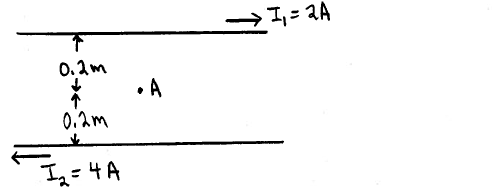# Problem: Two long straight parallel wires carry currents as shown in the sketch. Point  A is midway between the two wires, 0.20 m from each wire. What are the magnitude and direction of the net magnetic field at point A due to the two wires?

###### FREE Expert Solution
93% (438 ratings)
###### Problem Details

Two long straight parallel wires carry currents as shown in the sketch. Point  A is midway between the two wires, 0.20 m from each wire. What are the magnitude and direction of the net magnetic field at point A due to the two wires?